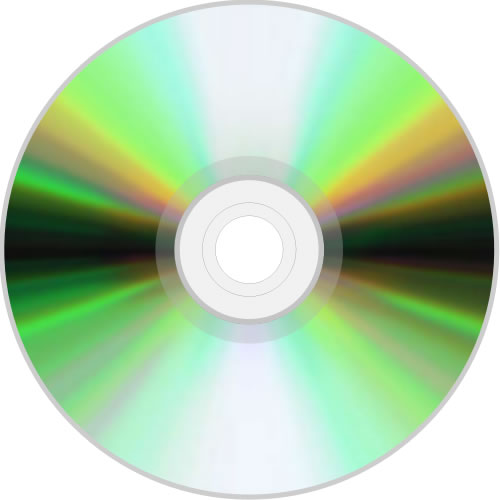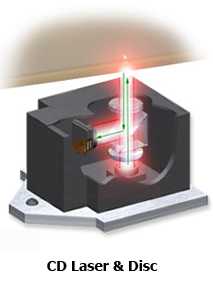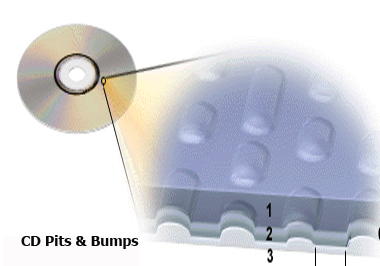< previous - 1 - 2 - 3 - 4 - next >

# Sound Reproduction1982 Digital Audio Launch

##The compact disc launched the digital audio revolution in 1982.  CD fabrication begins with the voltage produced by a microphone and captured/recorded by a magnetic tape, hard drive, or solid-state drive.

The amplitude of the microphone's voltage is sampled, looked at and measured, 44,100 times per second by an analog to digital converter, a sampling rate of 44.1KHz.  The sampled voltage is compared to and assigned a value from a predetermined table of 65,536 values.  The assigned-value is a close approximation of the originally sampled-voltage.

Each value is a 16-bit binary word from a numeric alphabet of two numbers, 0 & 1, defined as bits.  The binary word is simply a string of 16 zeros or ones with a total of 65,536 possible permutations.  Each bit location in the string represents a fixed percentage of the total possible voltage.  Each bit in the string has its particular voltage turned on or off per its instructions: 0 = Off  & 1 = On.

All 16 bits in the ON position = total possible voltage.
The 1st bit in the string = half of the total possible voltage.
The 2nd bit = half of the 1st bit.
The 3rd bit  = half of the 2nd bit.
The 4th bit  = half of the 3rd bit.
This pattern continues to the 16th bit.

As an example:
1111111111111111 = the total possible voltage.
00000000000 = 0 volts.
10000000000  = 1/2 of the total voltage.
01000000000  = 1/4 of the total voltage.
11000000000  = 3/4 of the total voltage.

Handbook Note:
A micron or micrometer = one millionth of a meter.

The 16-bit CD data embeds as a microscopic 0.5-micron wide spiral track of injected pits onto the poly-carbonate molded compact disc surface that begins near the center of the disc.  The injected-pits, covered in a reflective aluminum layer, are protected by a top acrylic layer.  A laser targets the spiral track during playback as the disc spins. The CD-pits and the area between the pits alter the reflecting laser light.  An optoelectric pickup converts the reflected light into an electric current.

A digital to analog converter samples the voltage 44.1 thousand times per second as it translates the 16-bit code.  A sampled change created by a land-to-pit or pit-to-land equals a digital 1 ON-command.  No change per sampled look equals a digital 0 OFF-command.  The converter reconstructs the 16-bit words and produces the assigned voltages.  The voltage total is amplified and delivered to the loudspeaker.Handbook Notes:
CD is a 16-bit code sampled at 44.1 thousand times per second.
DVD is a 24-bit code sampled at 96 thousand time per second.
DVD-A is 24-bit code sampled at 192 thousand times per second.
Blu-Ray is a 24-bit code sampled at 192 thousand times/second with up to 6 audio channels, or 92 thousand time/second with up to 8 audio channels.

< previous - 1 - 2 - 3 - 4 - next >

Ed's AV Handbook